## 泉州一中开放日4.21--体验趣味编程

### 认识GoC基本命令

 GoC基本命令（只能用小写字母，pen可以简写成p） 前进：pen.fd(步数); 提笔：pen.up(); 笔颜色：pen.c(0-15); 后退：pen.bk(步数); 落笔：pen.down(); 笔粗:pen.size(1-10); 左转：pen.lt(度数); 藏笔：pen.hide(); 复位：pen.move(0,0); 右转:pen.rt(度数); 显笔：pen.show(); 清屏：pen.cls(0-15);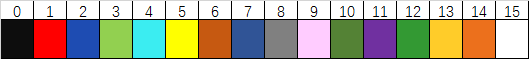运行结果：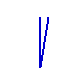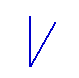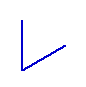实现代码： ```int main() { p.bk(50); p.rt(10); p.fd(50); return 0; }``` ```int main() { p.bk(50); p.rt(30); p.fd(50); return 0; }``` ```int main() { p.bk(50); p.rt(60); p.fd(50); return 0; }``` 运行结果：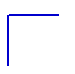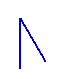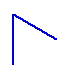实现代码： ```int main() { p.fd(50); p.rt(90); p.fd(50); return 0; }``` ```int main() { p.fd(50); p.rt(180-30); p.fd(50); return 0; }``` ```int main() { p.fd(50); p.rt(120); p.fd(50); return 0; }```

①运行“8个8边型.cpp”,出现运行结果：

 ```int main() { int n=8; for(int i = 0;i < n;i++) { for(int j = 0;j < n;j++) pen.fd(50).rt(360.0/n); pen.rt(360.0/n); } return 0; }```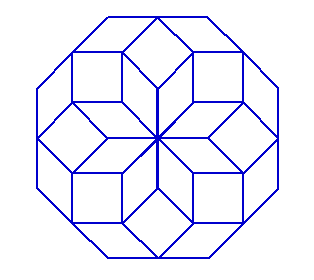②按一下键盘上的印刷屏幕键Printscreen或者PrtScr，截图复制到画图软件（开始-程序-附件-画图-粘贴），填充不同的颜色得到不同的图案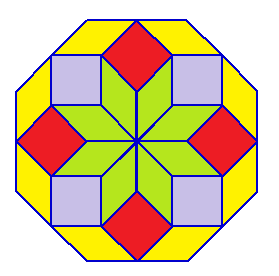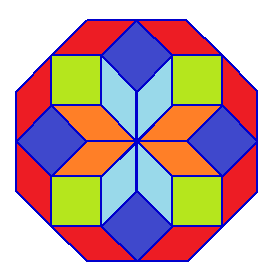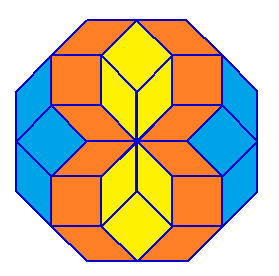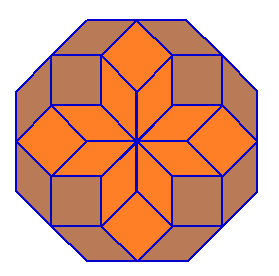③把int n=8；中的数字8改成6、10……，程序运行后按Printscreen键截图复制到画图软件，填充不同的颜色得到不同的图案：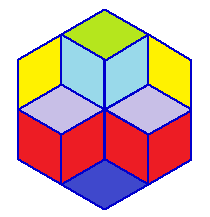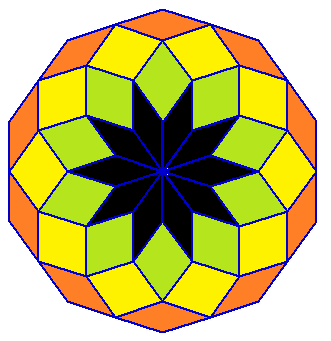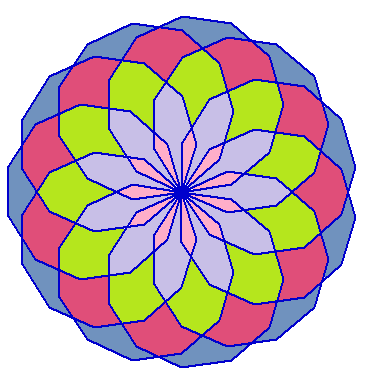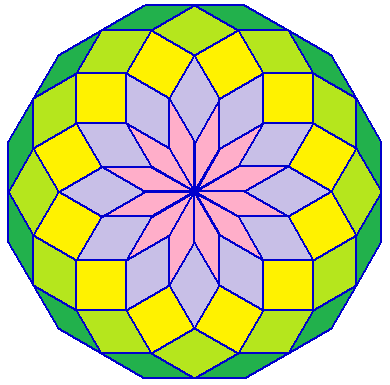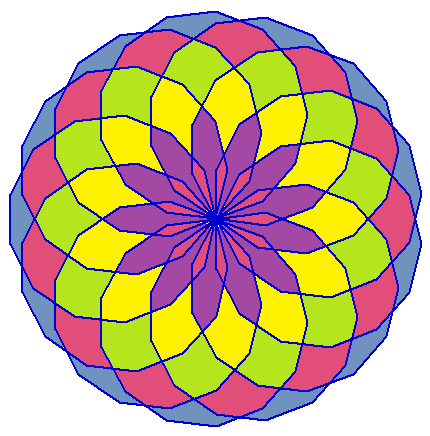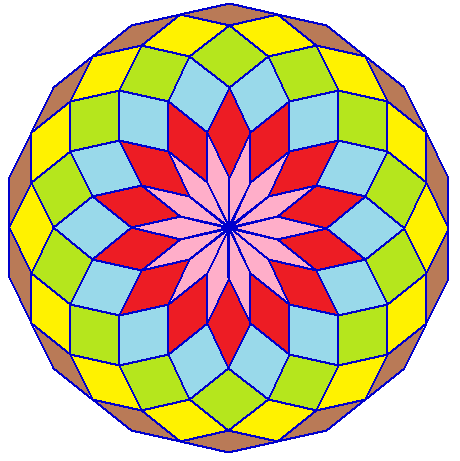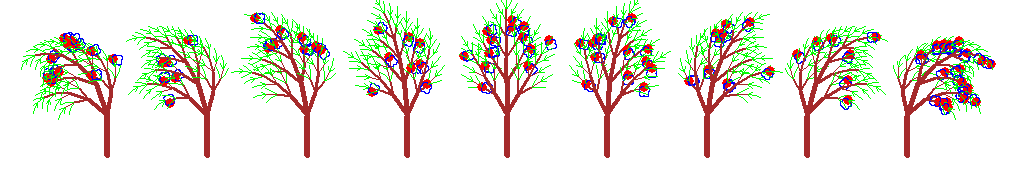```void hua(float a,long b)
{
for(int i=1;i<=4;i++)
{
for(int j=1;j<=180;j++)
p.fd(a).rt(b);p.lt(90);
}
}
void tree(int n,int f)
{
if(n<5)return ;
int s=rand()%100;
if(n<10)
{
if(s%20==0)p.oo(5,1);
if(s%20==0)
{
p.c(2);
hua(0.05,1);
}
p.c(3);
}
else  p.c(6);
pen.size(n/6).fd(n);
p.lt(30-f);
tree(n*0.6,f);
p.rt(30);
tree(n*0.8,f);
p.rt(30);
tree(n*0.6,f);
if(n<10)p.c(3);
else p.c(6);
p.lt(30+f).bk(n);
}
int main()
{
p.move(-400,0).angle(0);tree(40,-20);
p.move(-300,0).angle(0);tree(40,-15);
p.move(-200,0).angle(0);tree(40,-10);
p.move(-100,0).angle(0);tree(40,-5);
p.move(0,0).angle(0);tree(40,0);
p.move(100,0).angle(0);tree(40,5);
p.move(200,0).angle(0);tree(40,10);
p.move(300,0).angle(0);tree(40,15);
p.move(400,0).angle(0);tree(40,20);
return 0;
}```

……